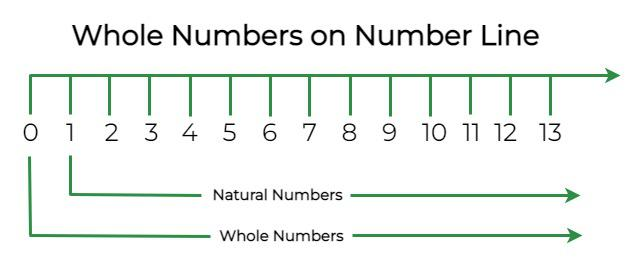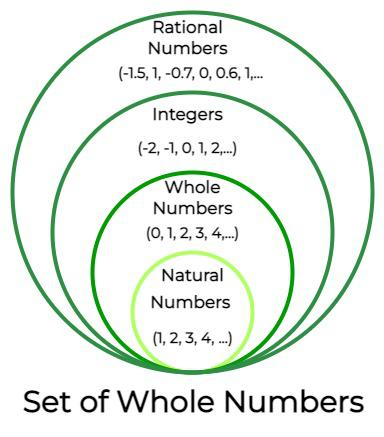Open in App
Not now

# Whole Numbers

• Last Updated : 06 Sep, 2022

Whole numbers are defined as the natural numbers on the number line, including zero. Complex numbers have two parts; they are real and imaginary numbers. Whole numbers are a part of the real numbers, excluding decimal numbers, fractions, and negative numbers. Numbers generating from 0 and going up to infinity are whole numbers. Let’s learn in detail about whole numbers, along with examples.

## What are Whole Numbers?

Whole numbers are the natural numbers starting with 0. The numbers 0, 1, 2, 3, 4, 5, 6, and (so on) are the whole numbers. It can be said that The whole number is the set of numbers without fractions, decimals, and negative numbers. It is a set of only positive numbers, including 0. The number smallest among the whole numbers is 0. Whole numbers are a part of real numbers just like natural numbers. However, the difference between whole numbers and natural numbers is that whole numbers contain all natural numbers and 0. This counts as the major difference between counting numbers and whole numbers. 0 is excluded in counting numbers.

### Whole Number Definition

In mathematics, whole numbers are defined as the set of natural numbers, including 0. If the set of natural numbers is represented as N, then N = {1, 2, 3, 4, …} and if the set of whole numbers is represented as W, then whole numbers in sets will be, W = {0, 1, 2, 3, 4, …}. Integers are a set of both positive and negative numbers, but whole numbers are only the positive set, including 0. Below are some points that should be kept in mind regarding whole numbers,

• All whole numbers come under real numbers.
• All natural numbers are whole numbers but not vice-versa.
• All positive integers, including 0, are whole numbers.

### Smallest Whole Number

0 is the smallest whole number. The definition of a whole number says that the whole number generates from 0 and goes up to ∞. Therefore, 0 becomes the smallest whole number that exists. 0 is neither positive nor negative; it is used as a placeholder.

### Whole Number Symbol

The symbol that represents whole number is “W”, W is the set for whole numbers, and the value in the set are the positive integers, including 0. W = {0, 1, 2, 3,…}.

## Whole Numbers on Number Line

Whole numbers on a number line can easily be observed as the number line, in the general case, represents integers from -∞ to +∞ , and whole numbers are positive integers, including 0. Looking at a number line, it can be observed that the integers lying on the right side of 0, including 0, are all whole numbers, and the integers lying on the right side of 0, excluding 0, are all natural numbers. This visual representation is the best way to conclude that all natural numbers are whole numbers but not vice versa.## Natural Numbers and Whole Numbers

Natural numbers and whole numbers are now known to us. It can be easily observed from the above number line that all natural numbers are whole numbers, the set of natural numbers is a subset of the whole numbers, and hence, the set of whole numbers W is the proper superset of the set of natural numbers N. Below diagram shows how the set of natural numbers, whole numbers, integers, rational numbers are related to each other.### Difference between Natural Numbers and Whole Numbers

Below is the table that contains the difference between natural numbers and whole numbers,

## Properties of Whole Numbers

The basic operation done in mathematics lead to the properties of whole number, that is, properties based on addition, subtraction, multiplication, and division. The main properties of whole numbers are:

• Closure Property
• Commutative Property
• Associative Property
• Distributive Property

### Closure Property

In this property, if x and y are the whole numbers, then x + y is a whole number, and xy is also the whole number. The statement for the closure property is that the sum and the product of two whole numbers will always be a whole number.

x + y = W

x × y = W

For example: Prove the closure property for 2 and 5.

2 is a whole number, and 5 is a whole number. To prove the closure property, add and multiply 2 and 5.

2 + 5 = 7 (Whole number).

2 × 5 = 10 (Whole number).

In the commutative property of addition, the sum of any two whole numbers is the same. i.e., the order of addition doesn’t matter. i.e.,

x + y = y + x

For example: Prove the commutative property of addition for 5 and 8.

According to the commutative property of addition:

x + y = y + x

5 + 8 = 13

8 + 5 = 13

Therefore, 5 + 8 = 8 + 5

### Commutative Property of Multiplication

In the commutative property of multiplication, the multiplication of any two whole numbers is the same. i.e., any number can be multiplied in any order. i.e.,

x × y = y × x

For example: Prove the commutative property of multiplication for 9 and 0.

According to the commutative property of multiplication:

x + y = y + x

9 × 0 = 0

0 × 9 = 0

Therefore, 9 × 0 = 0 × 9

In the additive property, When we add the value with zero, then the value of the integer remains unchanged. i.e.,

x + 0 = x

For example: Prove additive property for 7.

x + 0 = x

7 + 0 = 7

Hence, proved.

### Multiplicative Identity

In the multiplicative property, When we multiply the value by 1, then the value of the integer remains unchanged. i.e.,

x × 1 = x

For example: Prove multiplicative property for 13.

According to multiplicative property:

x × 1 = x

13 × 1 = 13

Hence, proved.

### Associative Property

In the associative property, when adding and multiplying the number and grouped together in any order, the value of the result remains the same. i.e.,

x + (y + z) = (x + y) + z

And

x × (y × z) = (x × y) ×  z

For example: Prove the associative property of multiplication for the whole numbers 10, 2, and 5.

According to the associative property of multiplication:

x × (y × z) = (x × y) ×  z

10 × (2 × 5) = (10 × 2) × 5

10 × 10 = 20 × 5

100 = 100

Hence, Proved.

### Distributive Property

In the distributive property, When multiplying the number and distributing them in any order, the value of the result remains the same. i.e.,

x × (y + z) = (x × y) + (x × z)

For example: Prove the distributive property for 3, 6, and 8.

According to the distributive property:

x × (y + z) = (x × y) + (x × z)

3 × (6 + 8) = (3 × 6) + (3 × 8)

3 × (14) = 18 + 24

42 = 42

Hence, Proved.

## Solved Examples on Whole Numbers

Example 1: Are the numbers 100, 399, and 457 the whole numbers?

Yes, the numbers 100, 399, 457 are the whole numbers.

Example 2: Solve the equation 15 × (10 + 5) using the distributive property.

We know that distributive property are:

x × (y + z) = x × y + x × z.

So, 15 × 10 + 15 × 5 = 150 + 75

= 225.

Example 3: Prove the associative property of multiplication for the whole numbers 1, 0, and 93.

Solution:

According to the associative property of multiplication:

x × (y × z) = (x × y) ×  z

1 × (0 × 93) = (1 × 0) × 93

1 × 0 = 0 × 93

0 = 0

Hence, Proved.

Example 4: Write down the number that does not belong to whole numbers:

4, 0, -99, 11.2, 45, 87.7, 53/4, 32.

Out of the numbers mentioned above, it can easily be observed that 4, 0, 45, and 32 belong to whole numbers. Therefore, the numbers that do not belong to whole numbers are -99, 11.2, 87.7, and 53/4.

Example 5: Write 3 whole numbers occurring just before 10001.

If the sequence of whole numbers are noticed, it can be observed that the whole numbers have a difference of 1 between any 2 numbers. Therefore, the whole numbers before 10001 will be: 10000, 9999, 9998.

Example 6: What is the mean of the first five whole numbers?

The mean of the first five whole numbers is:

First five whole numbers = 0, 1, 2, 3, 4

Mean of first five whole numbers = (0 + 1 + 2 + 3 + 4)/5

= 10/5

= 2

Therefore, the mean of the first five whole numbers is 2.

## FAQs on Whole Numbers

Question 1: Can a whole number ever be negative?

No, a whole number can never be negative as the set of whole numbers “W” is represented as:

W = {0, 1, 2, 3, …}

Therefore, whole numbers do not contain negative numbers.

Question 2: Are all Whole numbers Integers?

Yes, all whole numbers are integers but not vice-versa. Integers contain negative numbers and negative numbers are not a part of whole numbers.

Question 3: What are the symbols that are used to represent the set of natural numbers and whole numbers?

“W” is the symbol for the set of whole numbers, and “N” is the symbol for the set of natural numbers.

Question 4: How to multiply fractions with whole numbers?

Since whole numbers, if required to be represented in the form of a fraction, can be represented with 1 in the denominator. When a fraction is required to be multiplied by a whole number, simply multiply the numerator with the numerator and the denominator with the denominator.

For example 6 × 30/76

6/1 × 30/76

(6 × 30)/(1 × 76)

180/76 = 90/38 = 45/19

Question 5: 1 is the smallest whole number. True or False?

False. The smallest whole number is 0 as the whole number generates from 0 and terminates at ∞. Therefore, 1 is not the smallest whole number.

Question 6: Every integer is a whole number. Is the statement True or False?